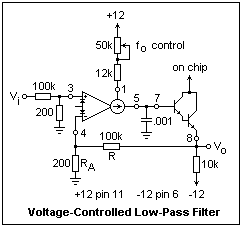## The Operational Transconductance Amplifier

Yet another op-amp-like device is the operational transconductance amplifier, or OTA, exemplified by the LM13600, a dual amplifier in a 16-pin DIP. It has inverting and noninverting inputs, and an output, like a a normal op-amp, but it is a very different device, and cannot be used like a voltage op-amp. Its applications are specialized, even more so than those of the Norton op-amp, which at least could be persuaded to do the things a normal op-amp does. The OTA is frequently used as an open-loop amplifier. Its gain is not high, and it is not useful in most of the familiar feedback circuits.

The idea of the OTA is to provide a transconductance amplifier, whose input is the differential voltage between its inputs, which are the bases of the transistors of a standard differential amplifier, and whose output is a current proportional to the differential voltage. The transconductance is the ratio of the output current to the input voltage, denoted by gm. The transconductance of an OTA is proportional to the bias current IABC established by an external resistor. At a bias current of 500 μA, the gm of the LM13600 is typically 9.6 mS, but can vary from 6.7 mS to 13.0 mS.

Because the full-scale range of the input differential voltage is quite small, less than a volt, compensation diodes are provided to linearize the input over a wider range by using the voltage drops across the diodes in parallel with the bases. These diodes are indicated on the circuit symbol for an OTA. We shall not use them here; for further information refer to the data sheets.

The difference in the collector currents of the transistors in the input differential amplifier is copied by three current mirrors within the chip and furnished to the output. There is also a Darlington buffer transistor on the chip, with the base and emitter connections brought out to pins. The collectors are internally connected to the positive supply. This buffer is usually necessary in the ouput circuit of the OTA.A circuit that demonstrates one use of an OTA is shown at the right, using an LM13600. Note the strange symbol for the OTA. Negative feedback is employed in this circuit, which is a low-pass filter whose corner frequency can be electrically adjusted. For the values shown, I obtained a filter that would tune from 2700 Hz to 9000 Hz, approximately. The gain is unity below the corner frequency, and rolls off at 20 dB/decade, a first-order response like an RC circuit. The feature is, of course, the electrical controllability of the corner frequency, which is here simulated by rotating a potentiometer. The 12k fixed resistor guarantees that you will not exceed the maximum 2 mA for the bias current. The voltage at pin 1 is -10.8 V in this circuit (two diode drops above the negative supply). The pin numbers for one of the amplifiers are shown, going down the left-hand side of the chip. The pins for the other amplifier are in the same order on the other side. Note the unusual location of the supply pins.

To analyze the circuit, we note that the output voltage is the reactive voltage drop across the capacitor driven by the current source, gm(1/jωC)(v+ - v-). Assume that the buffer Darlington has an infinite input resistance, so the amplifier drives only the capacitor. A fraction RA/(R + RA) of the output voltage is fed back to the inverting input. Now we can solve for v- in terms of v+, find the output voltage in terms of v+, and finally convert v+ to Vi with the voltage divider. The final result is Vo = Vi/[1 + jωC(R + RA)/ gmRA]. This is just a single-pole response with corner at fo = (gm/2πC)[RA / (R + RA)]. The corner frequency should be directly proportional to the bias current.

To test the circuit, first note the output peak-to-peak value at some low frequency, a few hundred Hz. Find the value 0.707 times this, and increase the frequency while watching the output (the input voltage should not vary) until it falls to this value. The corresponding frequency is the corner frequency. It is not hard to get four or five points this way, and to plot the corner frequency as a function of the bias current. My curve did not quite go through the origin, but I got a slope of 6.35 kHz/mA. You can also check the rolloff for some value of the bias current.

The OTA should not be confused with "programmable" op-amps (like the LM346), which are normal voltage op-amps whose operating current can be varied, or programmed, by an external resistor. This permits the current used to be the minimum possible in low-drain designs. The operating current affects many of the characteristics of the op-amp, but not the gain.